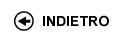Politecnico di Torino
01LQRDR, 01LQRAX, 01LQRJA
Probability and statistics
Corso di Laurea in Ingegneria Meccanica - Vercelli
Corso di Laurea in Ingegneria Civile - Vercelli
Corso di Laurea in Electronic And Computer Engineering - Vercelli
 Docente Qualifica Settore Lez Es Lab Tut Anni incarico Puppo Gabriella30 20 0 0 3
 SSD CFU Attivita' formative Ambiti disciplinari MAT/06 5 A - Di base Matematica, informatica e statistica
Esclusioni:
01BXT
 Obiettivi dell'insegnamento The course provides basic knowledge on statistics and probability theory. Competenze attese Ability to solve applied problems involving basic probability theory and to analyze data with simple tools from statistics. Prerequisiti Calculus I and II. Programma Probability. Introduction to probability and counting. Axioms of probability. Conditional probability and Bayes theorem. Discrete random variables: binomial and Poisson distributions. Expectation and distribution parameters. Continuous random variables: Gamma and Normal distributions. Joint distributions: covariance and correlation, linear regression. Statistics. Point estimation. Central limit theorem. Statistical intervals based on a single sample. Inferences on the mean and on variance. Testing hypotheses. Laboratori e/o esercitazioni Problem sessions will widen and deepen the topics treated in the lectures. Time will be also devoted to problem solving and working out examples and exercises. Bibliografia Main text: J.S. Milton, J.C. Arnold Introduction to Probability and Statistics: Principles and Applications for Engineering and the Computing Sciences, 4th edition Mc Graw Hill international editions Problem solving and exercises: J.S. Milton, J.C. Arnold Student Solutions Manual to accompany Introduction to Probability and Statistics, 4th Edition Mc Graw Hill international editions Controlli dell'apprendimento / Modalitŕ d'esame The exam will consist in a test requiring the solution of three or four exercises and in an oral discussion on the solution strategy used in the test. The written test will account for roughly 80% of the final grade. Orario delle lezioni Statistiche superamento esami Programma definitivo per l'A.A.2009/10